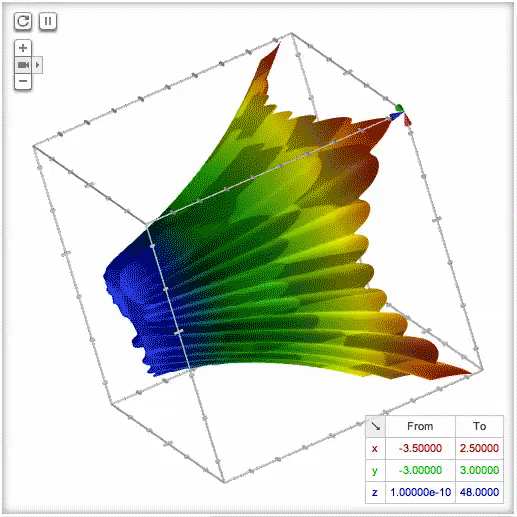In December Google released a graphing calculator in the search results. Google has just stepped that up by adding an animated and interactive version of their graphing calculator.

If you search for [sqrt(x*x+y*y)+3*cos(sqrt(x*x+y*y))+5 from -20 to 20], [sin(5.5x)*cos(5*y)+x*x+1 x is from -1 to 1, y is from -1 to 1, z is from 0.1 to 2.8], [tanh(y(y^4+5x^4-10(x^2)(y^2))/(x^2+y^2)^4)] or similar calculations, Google will now animated them and let you see them in 3D.

Here is a video:

Google shared this on their Google+ page and some note it is very similar to WolframAlpha - it is. But Google animated the graphs while Wolfram doesn't - well they do, but you have to pay for it.